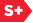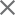Proceedings Paper

Density of Gabor Schauder bases
Author(s): Baiqiao Deng; Christopher E. Heil
Format Member Price Non-Member Price
PDF \$17.00 \$21.00

Paper Abstract

A Gabor system is a fixed set of time-frequency shifts G(g, (Lambda) ) equals [e2(pi ib x)g(x-a)] (a,b) (epsilon) (Lambda) of a function g (epsilon) L2(Rd). We prove that if G(g, (Lambda) ) forms a Schauder basis for L2(Rd) then the upper Beurling density of (Lambda) satisfies D+((Lambda) ) <EQ 1. We also prove that if G(g, (Lambda) ) forms a Schauder basis for L2(Rd) and if g lies in a the modulation space M1,1(Rd), which is a dense subset of L2(Rd), or if G(g, (Lambda) ) possesses at least a lower frame bound, then (Lambda) has uniform Beurling density D((Lambda) ) equals 1. We use related techniques to show that if g (epsilon) L1(Rd) then no collection [ g(x-a)]a (epsilon (Gamma) ) of pure translates of g can form a Schauder basis for L2(Rd). We also extend these results to the case of finitely many generating functions gl,...,gr.

Paper Details

Date Published: 4 December 2000
PDF: 12 pages
Proc. SPIE 4119, Wavelet Applications in Signal and Image Processing VIII, (4 December 2000); doi: 10.1117/12.408600
Show Author Affiliations
Baiqiao Deng, Columbus State Univ. (United States)
Christopher E. Heil, Georgia Institute of Technology (United States)

Published in SPIE Proceedings Vol. 4119:
Wavelet Applications in Signal and Image Processing VIII
Akram Aldroubi; Andrew F. Laine; Michael A. Unser, Editor(s)